Courses

# Test: Table Charts- 4

## 5 Questions MCQ Test General Aptitude for GATE 2020 | Test: Table Charts- 4

Description
This mock test of Test: Table Charts- 4 for LR helps you for every LR entrance exam. This contains 5 Multiple Choice Questions for LR Test: Table Charts- 4 (mcq) to study with solutions a complete question bank. The solved questions answers in this Test: Table Charts- 4 quiz give you a good mix of easy questions and tough questions. LR students definitely take this Test: Table Charts- 4 exercise for a better result in the exam. You can find other Test: Table Charts- 4 extra questions, long questions & short questions for LR on EduRev as well by searching above.
QUESTION: 1

###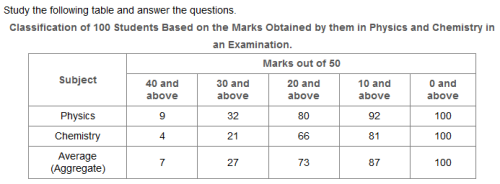What is the different between the number of students passed with 30 as cut-off marks in Chemistry and those passed with 30 as cut-off marks in aggregate?

Solution:

Required difference

= (No. of students scoring 30 and above marks in Chemistry)

- (Number of students scoring 30 and above marks in aggregate)

= 27 - 21

= 6.

QUESTION: 2

### Study the following table and answer the questions. Classification of 100 Students Based on the Marks Obtained by them in Physics and Chemistry in an Examination.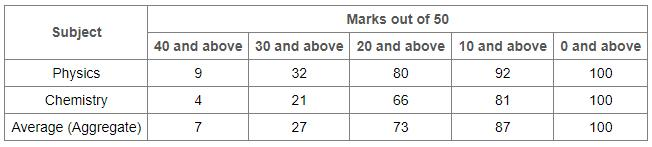If at least 60% marks in Physics are required for pursuing higher studies in Physics, how many students will be eligible to pursue higher studies in Physics?

Solution: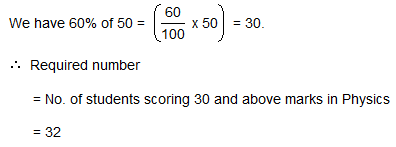QUESTION: 3

###The percentage of number of students getting at least 60% marks in Chemistry over those getting at least 40% marks in aggregate, is approximately?

Solution: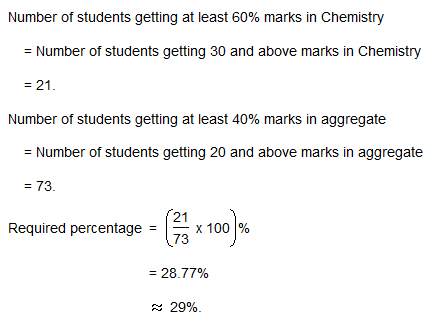QUESTION: 4The number of students scoring less than 40% marks in aggregate is?

Solution: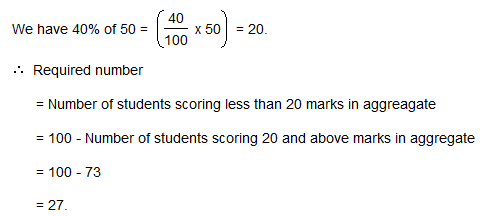QUESTION: 5If it is known that at least 23 students were eligible for a Symposium on Chemistry, then the minimum qualifying marks in Chemistry for eligibility to Symposium would lie in the range?

Solution:

The correct option is C.

It is clear from the above given table of classification of students. Here at least 23 students are eligible that means the marks range from 20-30 is best suitable.